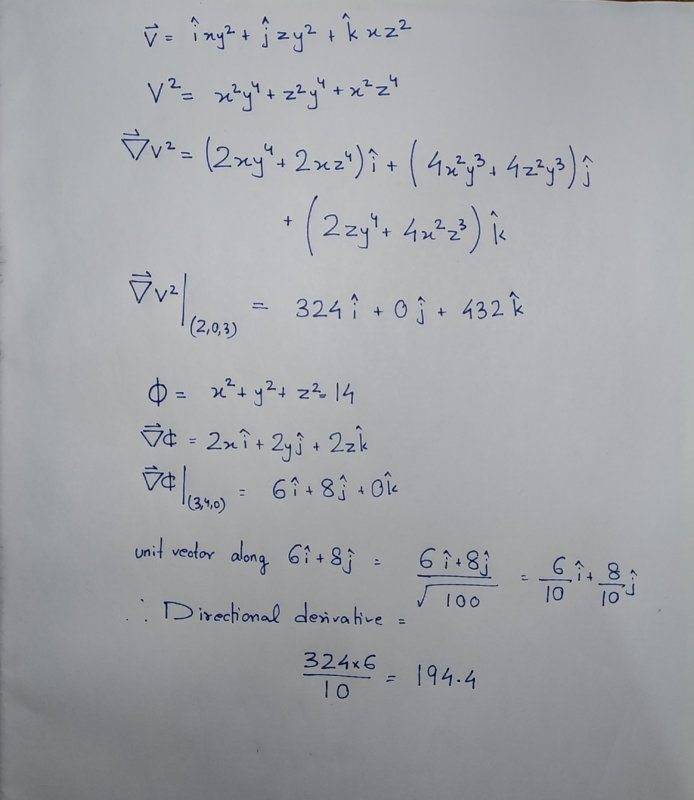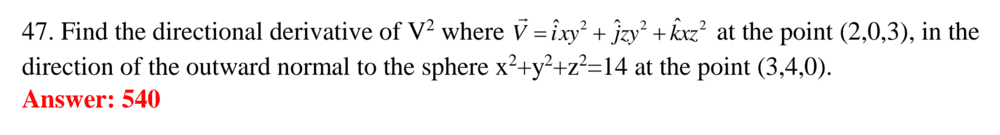# Finding the directional derivative

• Saptarshi Sarkar

#### Saptarshi Sarkar

Homework Statement
Find the directional derivative of ##V^2## where ##\vec V = \hat ixy^2 + \hat jzy^2 + \hat kxz^2## at the point (2,0,3), in the direction of the outward normal to the sphere ##x^2+y^2+z^2=14## at the point (3,4,0).
Relevant Equations
Directional Derivative = ##\nabla f.\hat n##
I tried to calculate the directional derivative but the answer that I found was 194.4 but the answer marked in the book was 540. I tried a lot but couldn't understand what my mistake was.
Please let me know what mistake I did.Is this the exact problem formulation?

Is this the exact problem formulation?

YesHomework Statement:: Find the directional derivative of ##V^2## where ##\vec V = \hat ixy^2 + \hat jzy^2 + \hat kxz^2## at the point (2,0,3), in the direction of the outward normal to the sphere ##x^2+y^2+z^2=14## at the point (3,4,0).
Relevant Equations:: Directional Derivative = ##\nabla f.\hat n##

I tried to calculate the directional derivative but the answer that I found was 194.4 but the answer marked in the book was 540. I tried a lot but couldn't understand what my mistake was.
Please let me know what mistake I did.

View attachment 256393

Notice what you obtain if you use (3, 0, 4) instead of the point (3, 4, 0) .

People working out answer keys have been known to make mistakes.

•PeroK, jim mcnamara and Saptarshi Sarkar

There's a peculiar randomness to this question. Note that the outward normal to a sphere (centred at the origin) is in the direction ##\hat r##. So, you could just write down the normal in this case: ##(\frac 3 5, \frac 4 5, 0)##, or otherwise.

•Saptarshi Sarkar
I'm a bit puzzled by the problem statement too, because ##(3,4,0)## isn't on the sphere ##x^2+y^2+z^2=14##... Could it be that the author wanted to consider the sphere ##x^2+y^2+z^2=25##?

••SammyS, PeroK and Saptarshi Sarkar
I'm a bit puzzled by the problem statement too, because ##(3,4,0)## isn't on the sphere ##x^2+y^2+z^2=14##... Could it be that the author wanted to consider the sphere ##x^2+y^2+z^2=25##?

It's very much possible. But even if (3,4,0) is not on the sphere, I can draw a normal to that point from the sphere's centre. But, most sphere equations that I see have a squared term on the RHS, so I think it was a typing mistake there.

The question is a normal to what? You need a surface to define a unit normal vector (in 3D) (up to a sign of course).

•Saptarshi Sarkar
Another thing to consider:

As O.P. determined, the gradient of V2 at the point (2, 0, 3) is:
##\displaystyle \left. \vec{\nabla} V^2 \right|_{(2,\ 0,\ 3)} = 324 \hat{i} + 0 \hat{j} + 432 \hat{k} ##

Now notice that the magnitude of that is 540 .

After reading the next post (#10, from @vanhees71 ) in this thread: I suppose that I should have been more complete in this post.

The thing to notice here is that the magnitude of gradient (the maximum directional derivative) is 540.

I was not trying to find the directional derivative along any normal to any surface.

Last edited:
Yes, but that's not a normal vector on the surface of the given sphere, because the point ##(2,0,3)## is not located at that sphere!

Yes, but that's not a normal vector on the surface of the given sphere, because the point ##(2,0,3)## is not located at that sphere!

These questions that the OP finds are often like this. First, there are two mistakes (perhaps typos) in the question: it should be ##25## instead of ##14##; and, the point on the sphere should be ##(3, 0, 4)##.

There's also the peculiar aspect that the normal to a sphere at a given point has nothing to do with the gradient of the given function at another point. It looks like two different questions "cobbled together".

•vanhees71
Ok, with the mentioned corrections the problem is well posed, and the solution is (at least conceptually) correct.